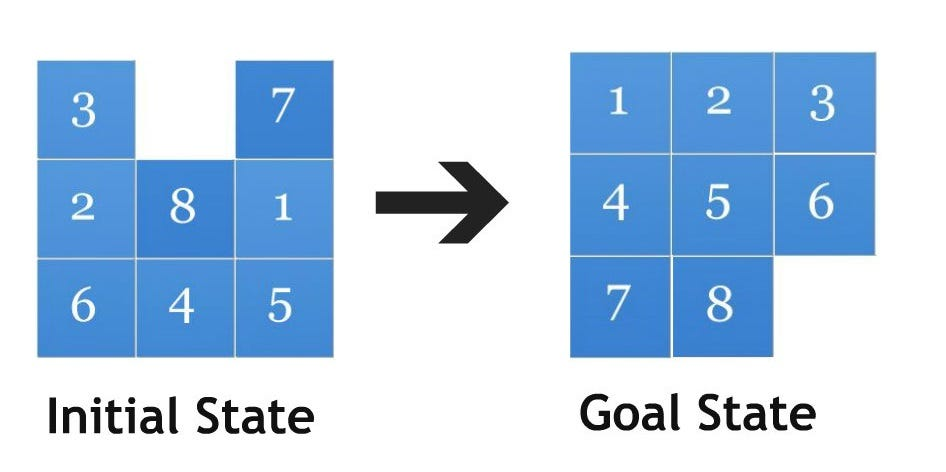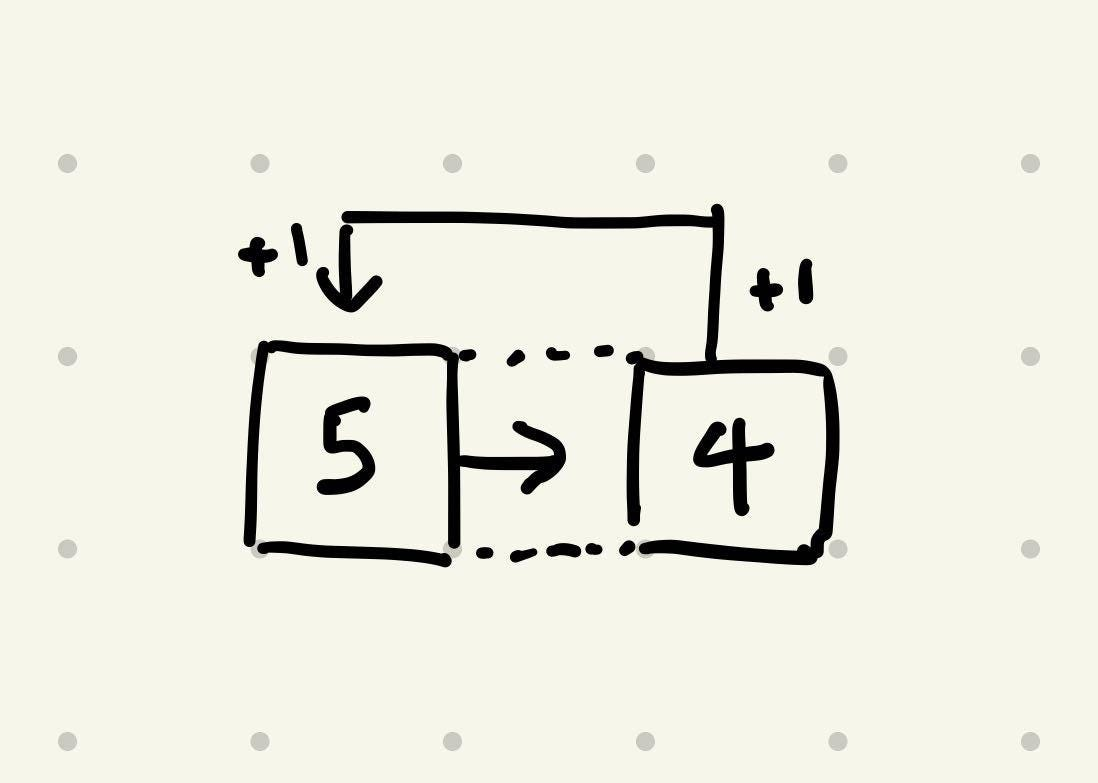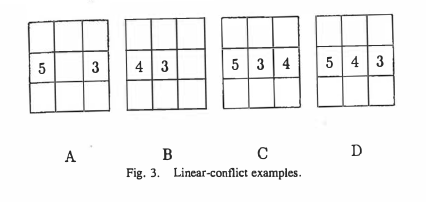# Looking into k-puzzle HeuristicsThe 8-puzzle is a simple sliding tile game where 8 tiles are jumbled in a 3 x 3 grid, and the player must slide tiles around to get the board into a “goal state”.

The k-puzzle is just a generalization of the 8-puzzle game, where k = n*n-1 and n is the width and height of the board.

The simplicity of the rules in contrast with the complexity of finding a solution makes it one of the quintessential application of A* search for introductory artificial intelligence courses. However, most courses seem to only introduce the basic heuristics to solve the problem, such as number of misplaced tiles, or manhattan distance, and more informed heuristics are not covered — implementations are even harder to come by. In this post, I attempt to explore and explain some of the heuristics that I’ve come across in my own research and found interesting.

## Linear Conflicts

2 tiles are in linear conflict if both tiles are in their target rows or columns, and in the same setting of the manhattan heuristic, they must pass over each other in order to reach their final goal position. Since we know that in an actual instance of a game, there is no possible way for tiles to actually slide over each other.

If such a conflict arises, then 1 of the tiles would need to move out of the aforementioned row or column, and back in again, adding 2 moves to the sum of their manhattan distances.Fig 2. Tiles 4 and 5 in linear conflictFig 3. Linear conflict examples from the original paper

To put it formally, 2 tiles tⱼ and tₖ are in linear conflict if tⱼ and tₖ are both in the same line, the goal positions of tⱼ and tₖ are both in that line, tⱼ is to the right of tₖ, and the goal position of tⱼ is to the left of the goal position of tₖ.

What happens when 3 or more tiles are in linear conflict?

Since an admissible heuristic makes an optimistic guess of the actual cost of solving the puzzle, we pick the tile involved in the most conflict to move out of the row (or column) first. This way we can minimize the number of additional moves.

### Implementation details

The linear conflict heuristic is easy to summarize into words, but hard to describe its implementation. The original pseudocode is here, but I will include the thought process behind my own implementation. Here goes.

Firstly, we can distill the problem to only find linear conflicts in a row. Once we are able to do this we can simply map this function on the overall grid to get all row conflicts of the given board.

``````def linear_conflicts(self, grid):
row_conflicts = [self.line_conflicts(row, i) for i, row in enumerate(grid)]
col_conflicts = [self.line_conflicts(col, i) for i, col in enumerate(self.transpose(grid))]
return sum(row_conflicts) + sum(col_conflicts)
def transpose(state):
width = len(state)
return [[state[j][i] for j in range(width)] for i in range(len(state))]
def line_conflicts(self, line, i):
.
.
.``````

For each tile in that row we loop through all other tiles to its right, and determine if there is an overlap in their paths. If the tile we are at has a target column of k, _and the tile we are comparing against as the target column of _j, then we are checking if j < k. _(Of course, there can never be the case where _j = k, since all tiles have a different goal position) Since the relationship of linear conflict is symmetric, we don’t have to check the left of the tile, since that case would have been considered in a previous iteration of this loop.

``````## main loop
for index, tile in enumerate(line):
if tile == 0:
continue
x, y = dest(tile)
if index != x:
## tile is not in target row
continue
for k in range(index + 1, self.n):
other_tile = line[k]
if other_tile == 0:
continue
tx, ty = dest(other_tile)
if tx == x and ty <= y:
## there is a pair in conflict!``````

Now we can start to view linear conflicts as a graph. For each pair of nodes that is involved in a linear conflict, we add both the forwards and backwards relationship of these 2 tiles into an adjacency list, forming an undirected conflict graph. Then we simply take the node with the largest degree and remove its occurrence in all of its neighbors, incrementing a counter by 1 each time. The process is completed once the graph is empty.

To extend this to column conflicts, we simply transpose the 2d array that represents our board, and run the same algorithm here. However, we need to take note that the target position indices must be flipped to account for the transposition, i.e., ((i, j) -> (j, i)). So, we modify the `line_conflict` function to take in a take in a function that gives us the appropriate goal position coordinates.

Here’s the full code if you wanna copy pasta.

``````## self.mapping is a hashtable of tile -> goal positions
## self.mapping = dict()
## for i, row in enumerate(self.goal_state):
##     for j, v in enumerate(row):
##         self.mapping[v] = (i, j)
def line_conflict(self, i, line, dest):
conflicts = 0
conflict_graph = {}
for j, u in enumerate(line):
if u == 0:
continue
x, y = dest(u)
if i != x:
continue

for k in range(j + 1, self.n):
## opposing tile
v = line[k]
if v == 0:
continue
tx, ty = dest(v)
if tx == x and ty <= y:
u_degree, u_nbrs = conflict_graph.get(u) or (0, set())
conflict_graph[u] = (u_degree + 1, u_nbrs)
v_degree, v_nbrs = conflict_graph.get(v) or (0, set())
conflict_graph[v] = (v_degree + 1, v_nbrs)
while sum([v for v in conflict_graph.values()]) > 0:
popped = max(conflict_graph.keys(),
key=lambda k: conflict_graph[k])
for neighbour in conflict_graph[popped]:
degree, vs = conflict_graph[neighbour]
vs.remove(popped)
conflict_graph[neighbour] = (degree - 1, vs)
conflicts += 1
conflict_graph.pop(popped)
return conflicts
def row_dest(self, v):
return self.mapping[v]                                                   def col_dest(self, v):
return self.row_dest(v)[::-1]``````

## AI Innovations in Artificial Intelligence

Innovations in Artificial Intelligence - Various sectors in which AI is remarkably used & has brought changes in humanity - Education, Healthcare,automobile

## Artificial Intelligence with Python | Artificial Intelligence Tutorial

"Artificial Intelligence With Python" will provide you with a comprehensive and detailed knowledge of Artificial Intelligence concepts with hands-on examples.

## Artificial Intelligence In 10 Minutes | What Is Artificial Intelligence?

You will learn AI in 10 minutes. You will understand a brief history of AI and look into what Artificial Intelligence is. Then you will see the types of AI and learn the different applications of AI. Finally, we'll see what the future of AI holds.

## Bursting the top 7 common Myths about Artificial Intelligence by Rebecca Harrison

Artificial Intelligence has been the go-to technology for companies and enterprises in recent years. The adoption of AI by enterprises all around the world has grown by 270% in the last four years a...

## 10 Most Amazing Artificial Intelligence Milestones To Know

Top 10 Artificial Intelligence Milestones to learn AI evolution - Origin,ELIZA,XCON,Statistics Introduction, Chess & jeopardy winner,autonomous vehicles